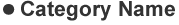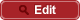• HOME
• RIKEN-QHP
• Evidence of a structure in $\bar{K}^0 \Lambda^+_c$ consistent with a charged $\Xi_c(2930)^+$, and updated measurement of $\bar{B}^0 \to \bar{K}^0 \Lambda^+_c \Lambda^-_c$ at Belle

##PrePrint No. RIKEN-QHP-395 Y. B. Li et al., (Belle Collaboration) including M. Takizawa Evidence of a structure in $\bar{K}^0 \Lambda^+_c$ consistent with a charged $\Xi_c(2930)^+$, and updated measurement of $\bar{B}^0 \to \bar{K}^0 \Lambda^+_c \Lambda^-_c$ at Belle arXiv: 1806.09182  (org) Eur. Phys. J. C 78, 928 (2018) 2018.06